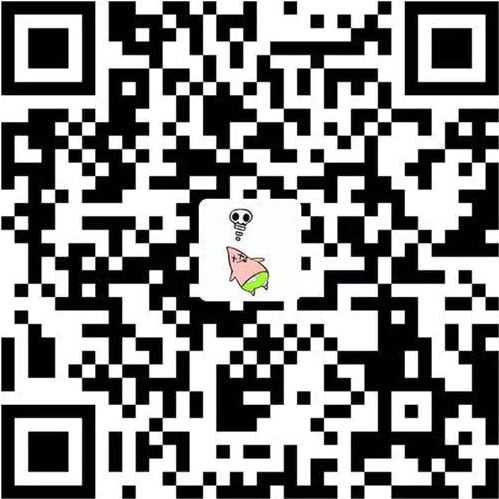# 657. 机器人能否返回原点

``````class Solution {

public boolean judgeCircle(String moves) {
int x = 0;
int y = 0;
for (int i = 0; i < moves.length(); i++) {
switch (moves.charAt(i)) {
case 'R':
x++;
break;
case 'L':
x--;
break;
case 'U':
y++;
break;
case 'D':
y--;
break;
}
}
return x == 0 && y == 0;
}

}
``````